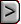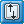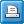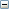﻿ CHOB - Specified-Head Flow Observation Package
Online Guide to MODFLOW-2000

CHOB - Specified-Head Flow Observation PackageCHOB - Specified-Head Flow Observation PackageCHOB - Specified-Head Flow Observation PackageAbbreviation in Name file

CHOB

Purpose

The Specified-Head Flow Observation input file is used to specify observations of flow through constant-head boundaries for use in the Observation process.

Documentation

 • Hill, M.C., Banta, E.R., Harbaugh, A.W., and Anderman, E.R., 2000, MODFLOW-2000, the U.S. Geological Survey modular ground-water model -- User guide to the Observation, Sensitivity, and Parameter-Estimation Processes and three post-processing programs: U.S. Geological Survey Open-File Report 00-184, 210 p.
 • release.txt
 • OBS.pdf

Related Packages

 • Observation Process input files,
 • Basic package,
 • Flow and Head Boundary package

Supported in

 • MODFLOW-2000
 • MODFLOW-2005
 • MODFLOW-LGR
 • MODFLOW-CFP
 • MODFLOW-NWT
 • MODFLOW-OWHM

Input instructions

Input for the Specified-Head Flow Observation input file is read from a file that is specified with "CHOB" as the file type listed in the name file.

Data Set 0

[#Text]

Item 0 is optional and can include as many lines as desired. Each line needs to begin with the “#” character in the first column.Text
 Text—is a character string (maximum of 79 characters) that starts in column 2. Any characters can be included in Text. The “#” character needs to be in column 1. Text is printed when the file is read and provides an opportunity for the user to include information about the model both in the input file and the associated output file.

Data Set 1

 NQCH NQCCH NQTCH (free format)Explanation of variablesNQCH
 NQCH—is the number of cell groups for which constant-head flow observations are listed. A group consists of the cells needed to represent one flow measurement (eq. 9).NQCCH
 NQCCH—is greater than or equal to the total number of cells in all groups. NQCCH must be greater than or equal to the sum of all |NQCLCH|.NQTCH
 NQTCH—is the total number of constant-head flow observations for all cell groups. NQTCH must equal the sum of all NQOBCH, which are specified in repetitions of item 3 in the input file.

Data Set 2

 TOMULTCH  EVFCH IOWTQCH (free format)Explanation of variablesTOMULTCH
 TOMULTCH—is the time-offset multiplier for constant-head flow observations [-- or T/T]. The product of TOMULTCH and TOFFSET must produce a time value with units that are consistent with the other model input. TOMULTCH can be dimensionless or can be used to convert the units of TOFFSET to the time unit used in the simulation.EVFCH
 EVFCH— is the error variance multiplier for constant-head flow observations, and is used to calculate the weights as described below in the explanation of STATISTIC. EVFCH makes it easy to change the weights uniformly for all constant-head flow observations.IOWTQCH
 IOWTQCH—is a flag that indicates that the variance-covariance matrix on constant-head flow observations used to calculate the weighting is to be read into array WTQ. If IOWTQCH equals zero, weights are assigned using STATISTIC of item 4; if it is greater than zero, items 6 and 7 are read.

Read items 3, 4, and 5 for each of NQCH groups of cells for which constant-head flow observations are to be specified.

Data Set 3

NQOBCH NQCLCH (free format)Explanation of variablesNQOBCH
 NQOBCH—is the number of times at which flows are observed for the group of constant-head cells.NQCLCH
 NQCLCH—is a flag, and the absolute value of NQCLCH is the number of cells in the group. If NQCLCH is less than zero, FACTOR = 1.0 for all cells in the group.

Data Set 4

 OBSNAM IREFSP TOFFSET HOBS STATISTIC STAT-FLAG PLOT-SYMBOL (free format)

Read item 4 for each of NQOBCH observation times for this group of cells. STATISTIC and STAT-FLAG are ignored if IOWTQCH is greater than zero.Explanation of variablesOBSNAM
 OBSNAM—is a string of 1 to 12 nonblank characters used to identify the observation.  The current version of MODFLOW-2000 (v 1.17.02) accepts duplicate observation names, but if duplicate names are found, a warning concerning the duplication is written to the Global file.IREFSP
 IREFSP—is the reference stress period to which the observation time is referenced. The reference point is the beginning of this stress period.TOFFSET
 TOFFSET—is the time offset of the observation, from the beginning of stress period IREFSP [T]. TOFFSET must be in units such that the product of TOMULTCH and TOFFSET is in time units consistent with other model input. TOFFSET and TOMULTCH from the CHOB file and values of PERLEN, NSTP, and TSMULT from the Discretization file (Harbaugh and others, 2000) are used to determine the stress period, time step, and time during the time step for the observation. To specify that an observation is for a steady-state model solution, specify IREFSP as the stress-period number of the steady-state stress period, and specify TOFFSET such that the product TOMULTCH * TOFFSET is less than or equal to PERLEN for the stress period; if PERLEN is zero, set TOFFSET to zero. If the observation falls within a time step, the simulated equivalent is calculated by linearly interpolating between values for the beginning and end of the time step. If the first stress period is transient and the observation falls within the first time step, the simulated equivalent from the end of the time step is used because no flow from the beginning of the time step is available for interpolation.HOBS
 HOBS—is the observed constant-head flow into (positive) or out of (negative) the system [L3/T].STATISTIC
 STATISTIC—is the value from which the weight for the observation is calculated, as determined using STAT-FLAG. STATISTIC is ignored if IOWTQCH is greater than zero, in which case WTQ of item 7 is used to define the weighting.STAT-FLAG

STAT-FLAG—is a flag identifying what STATISTIC is and how the weight is calculated.

 • STAT-FLAG is ignored if IOWTQCH is greater than zero.
 • STAT-FLAG = 0, STATISTIC is a scaled variance [(L3/T)2], weight = 1/(STATISTIC * EVFCH),
 • STAT-FLAG = 1, STATISTIC is a scaled standard deviation [L3/T], weight = 1/(STATISTIC2* EVFCH), and
 • STAT-FLAG = 2, STATISTIC is a scaled coefficient of variation [--], weight = 1/[(STATISTIC * HOBS)2* EVFCH].PLOT-SYMBOL
 PLOT-SYMBOL—is an integer that will be written to output files intended for graphical analysis to allow control of the symbols used when plotting data.

Data Set 5

LAYER ROW COLUMN FACTOR (free format)

Read item 5 for each of |NQCLCH| cells in this group.Explanation of variablesLAYER
 LAYER—is the layer index of a constant-head cell included in the cell group.ROW
 ROW—is the row index of a constant-head cell included in the cell group.COLUMN
 COLUMN—is the column index of a constant-head cell included in the cell group.FACTOR
 FACTOR—is the portion of the simulated flow for the cell that is included in the total simulated flow for this cell group (fn of eq. 16).

Read items 6 and 7 if IOWTQCH is greater than 0.

Data Set 6

FMTIN IPRN (free format)Explanation of variablesFMTIN
 FMTIN—is the Fortran format to be used in reading each line of the variance-covariance matrix used to calculate the weighting. The format needs to be enclosed in parentheses and needs to accommodate real numbers.IPRN

IPRN—is a flag identifying the format in which the variance-covariance matrix is printed. If IPRN is less than zero, the matrix is not printed. Permissible values of IPRN and corresponding formats are:

 Output requires more than 80 columns Output requires 80 columns or less IPRN FORMAT IPRN FORMAT 1 10G12.3 6 5G12.3 2 10G12.4 7 5G12.4 3 9G12.5 8 5G12.5 4 8G13.6 9 4G13.6 5 8G14.7 10 4G14.7

Data Set 7

WTQ(1,1), WTQ(1,2), WTQ(1,3), ... , WTQ(1,NQTCH) (format: FMTIN)

WTQ(2,1), WTQ(2,2), WTQ(2,3), ... , WTQ(2,NQTCH)

...

WTQ(NQTCH,1), WTQ(NQTCH,2), WTQ(NQTCH,3), ... , WTQ(NQTCH,NQTCH)WTQ
 WTQ—is an NQTCH by NQTCH array containing the variance-covariance matrix on constant-head flow observations [(L3/T)2]. For elements WTQ(I,J), if I ≠ J, WTQ(I,J) is the covariance between observations I and J; if I = J, WTQ(I,J) is the variance of observation I. The variance-covariance matrix is symmetric, but the entire matrix (upper and lower parts) must be entered.